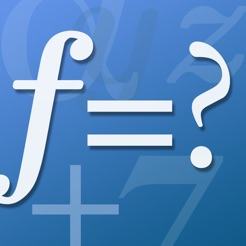## Capturas de ecrã

•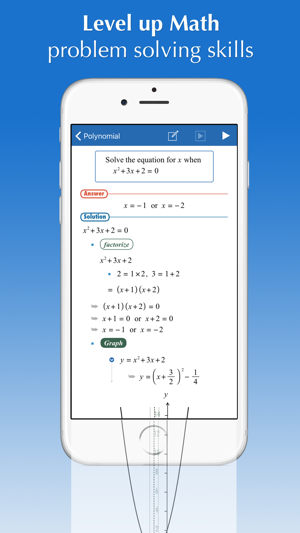••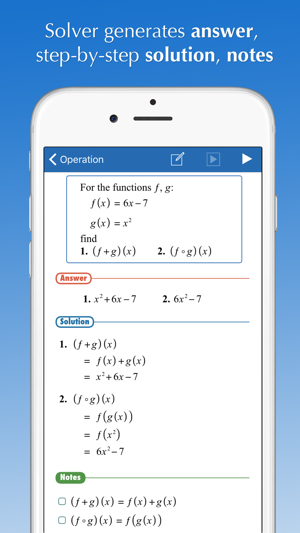•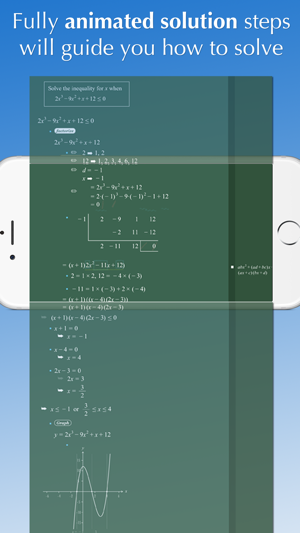•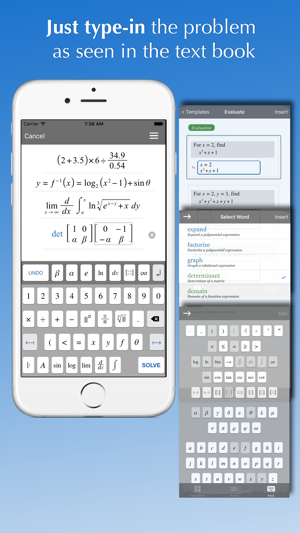## Descrição

Will guide you how to solve your math homework and textbook problems, anytime, anywhere.

FX Math Solver is a comprehensive math software, based on an automatic mathematical problem solving engine,
and ideal for students preparing term math exams, ACT, SAT, and GRE:

- Over 1,500 sample math problems and fully animated solution steps
- Scientific calculator supported
- Graphing calculator supported
- Automatic problem solving and generation of fully animated step-by-step procedures for problems typed in by users
- User friendly math problem expression editor (WYSIWYG mode)

Math Solver covers problems at the level of Pre-Algebra, Algebra1, Algebra2, and Calculus courses:

- Number operations: add, subtract, multiply, divide
- Prime factorization
- Mixed number
- Complex number

- Basic expression simplification:
- polynomial - factorization, long-division
- rational
- exponential and log/ln
- sin, cos, tan, ..
- Matrix
- Equations, system of equations
- Inequalities

- Function

- Graphing

- Limit

- Differentiation

- Integral

Versão 7.0

- Support iPhoneX UI
- Fix bug in solution animation player for iOS 12.0
- Fix bug in InApp purchase receipt check

4.5 de 5
2 classificações

2 classificações

## Informação

Fornecedor
Euclidus Inc
Tamanho
42.2 MB
Categoria
Ensino

Requer o iOS 11.0 ou posterior. Compatível com iPhone, iPad e iPod touch.

Idiomas

Inglês

Classificação de 4+
Preço
Grátis
•